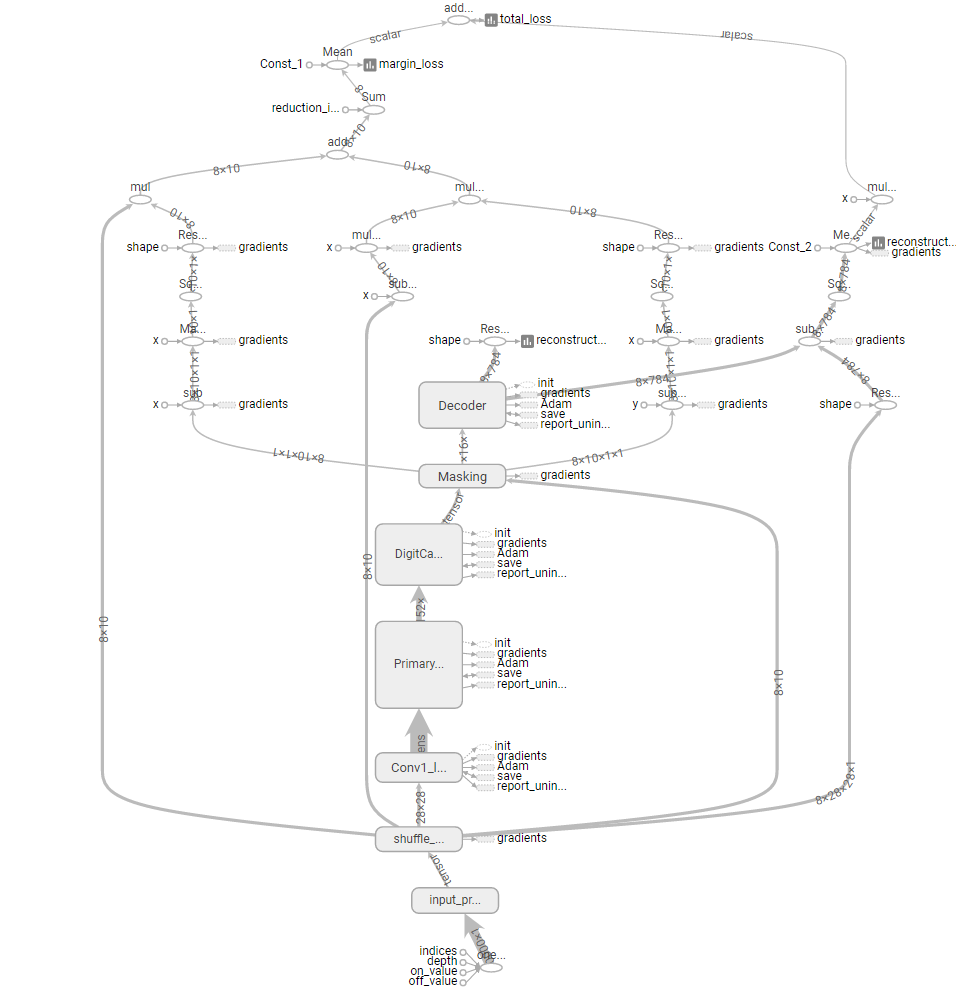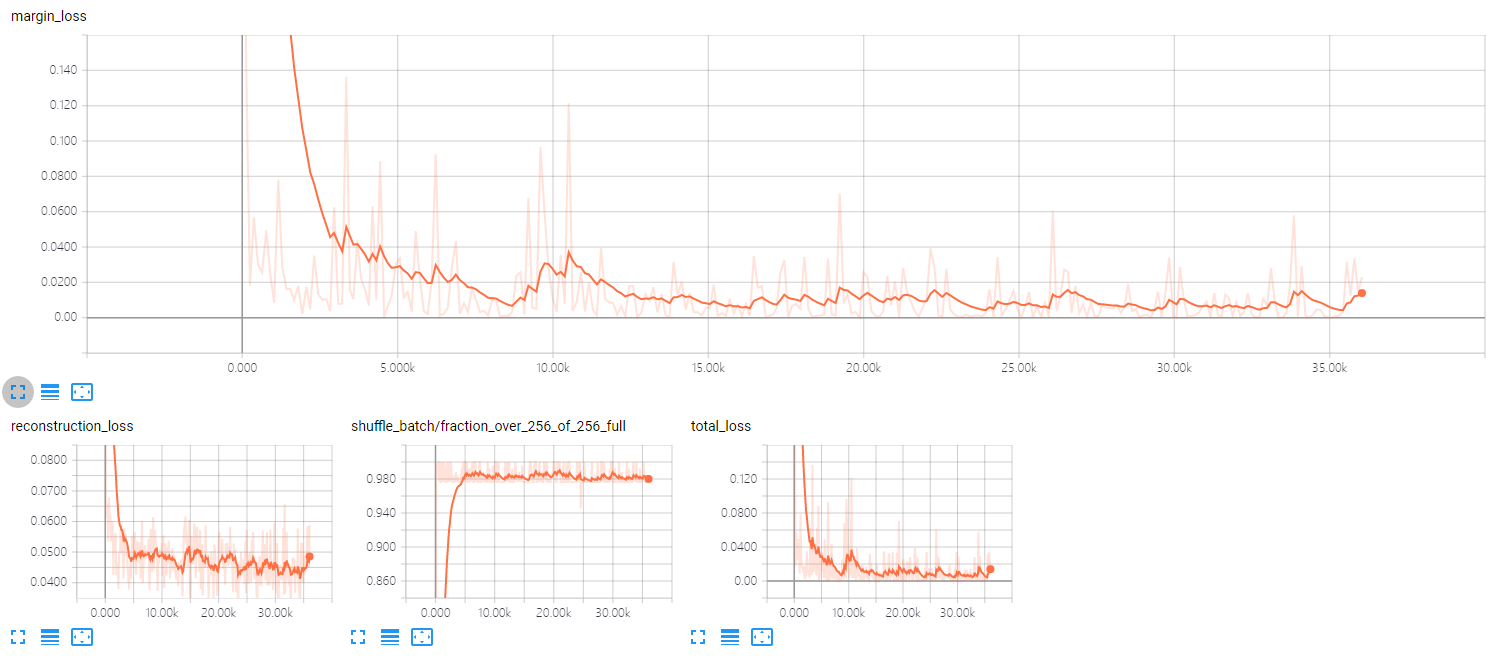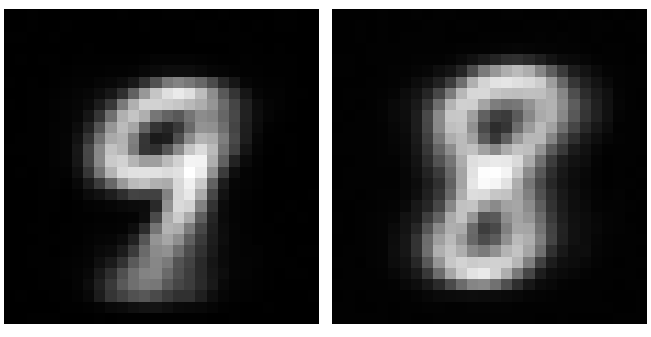# CapsNet

## 从CapsNet架构到TensorFlow实现：解析Hinton等人提出的Capsule

### 卷积层与卷积机制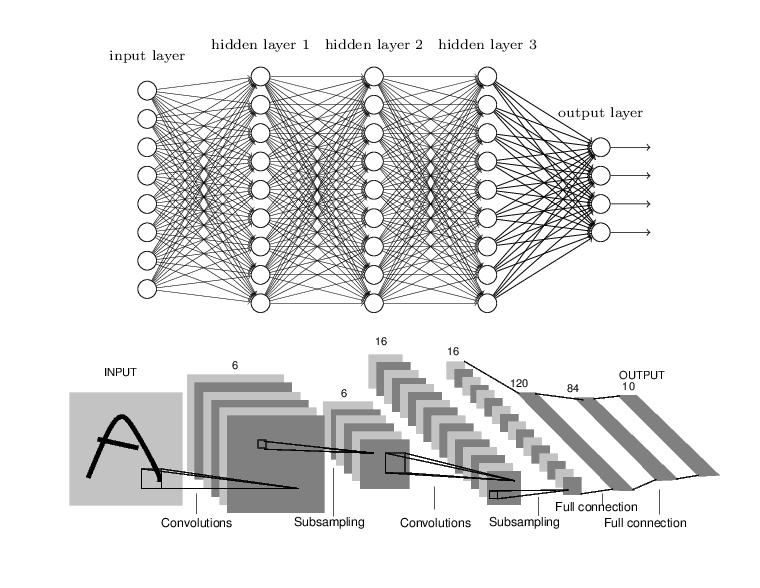#### 卷积层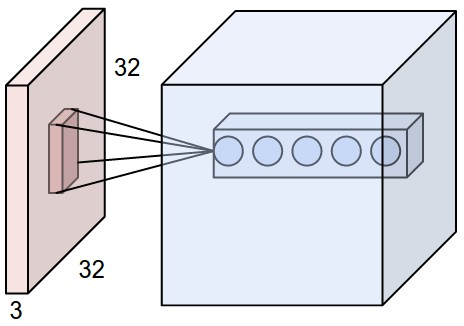#### 卷积操作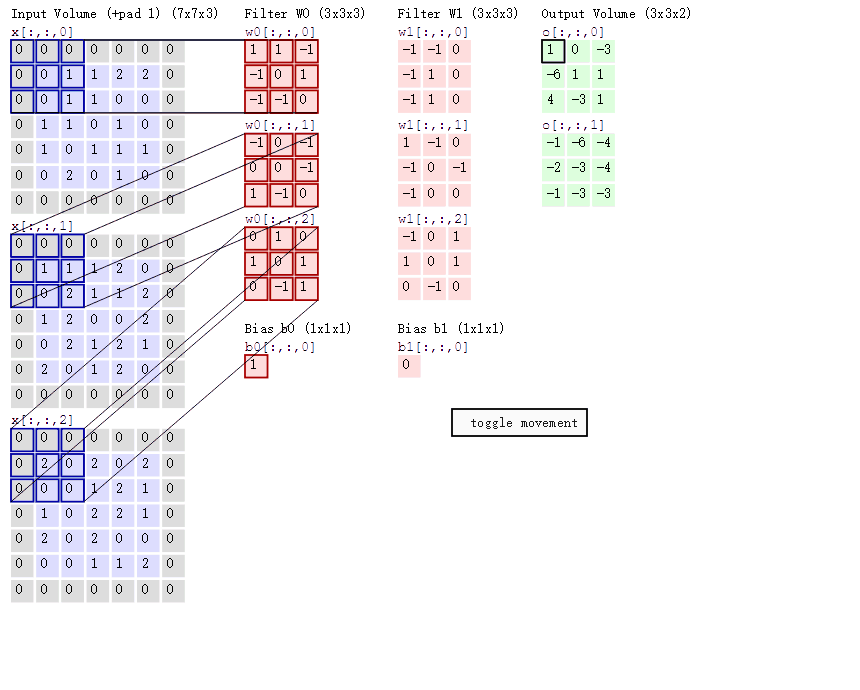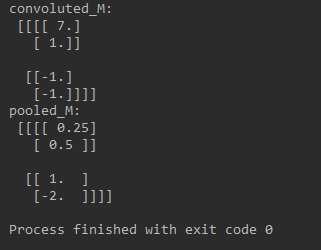### Capsule 层与动态路由

#### Capsule 层

Capsule 中的神经元的激活情况表示了图像中存在的特定实体的各种性质。这些性质可以包含很多种不同的参数，例如姿势（位置，大小，方向）、变形、速度、反射率，色彩、纹理等等。而输入输出向量的长度表示了某个实体出现的概率，所以它的值必须在 0 到 1 之间。

$\mathbf { v } _ { j } = \frac { | s _ { j } | ^ { 2} } { 1+ | \mathbf { s } _ { j } | ^ { 2} } \frac { s _ { j } } { | s _ { j } | }$

$\mathbf { s } _ { j } = \sum _ { i } c _ { i j } \hat { \mathbf { u } } _ { j | i } ,\quad \hat { \mathbf { u } } _ { j | i } = \mathbf { W } _ { i j } \mathbf { u } _ { i }$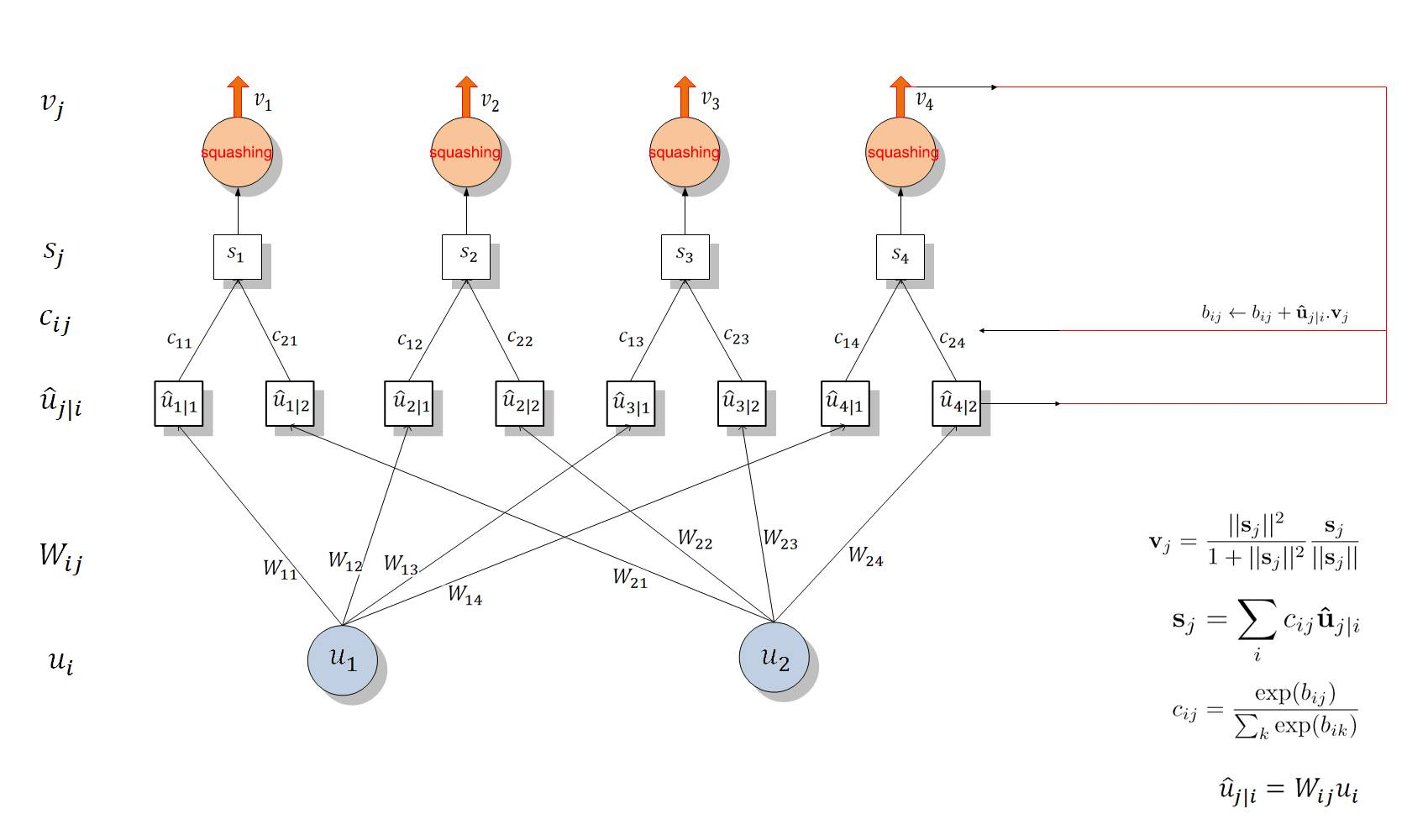#### Dynamic Routing 算法

$c _ { i j } = \frac { \exp \left( b _ { i j } \right) } { \sum _ { k } \exp \left( b _ { i k } \right) }$

b_ij 依赖于两个 Capsule 的位置与类型，但不依赖于当前的输入图像。我们可以通过测量后面层级中每一个 Capsule j 的当前输出 v_j 和 前面层级 Capsule i 的预测向量间的一致性，然后借助该测量的一致性迭代地更新耦合系数。本论文简单地通过内积度量这种一致性，即 $a _ { i j } = \mathbf { V } j \cdot \hat { \mathbf { u } } j | i$ ，这一部分也就涉及到使用 Routing 更新耦合系数。

Routing 过程就是上图 4 右边表述的更新过程，我们会计算 v_j 与 u_j|i hat 的乘积并将它与原来的 b_ij 相加而更新 b_ij，然后利用 softmax(b_ij) 更新 c_ij 而进一步修正了后一层的 Capsule 输入 s_j。当输出新的 v_j 后又可以迭代地更新 c_ij，这样我们不需要反向传播而直接通过计算输入与输出的一致性更新参数。### CapsNet 架构

Hinton 等人实现了一个简单的 CapsNet 架构，该架构由两个卷积层和一个全连接层组成，其中第一个为一般的卷积层，第二个卷积相当于为 Capsule 层做准备，并且该层的输出为向量，所以它的维度要比一般的卷积层再高一个维度。最后就是通过向量的输入与 Routing 过程等构建出 10 个 v_j 向量，每一个向量的长度都直接表示某个类别的概率。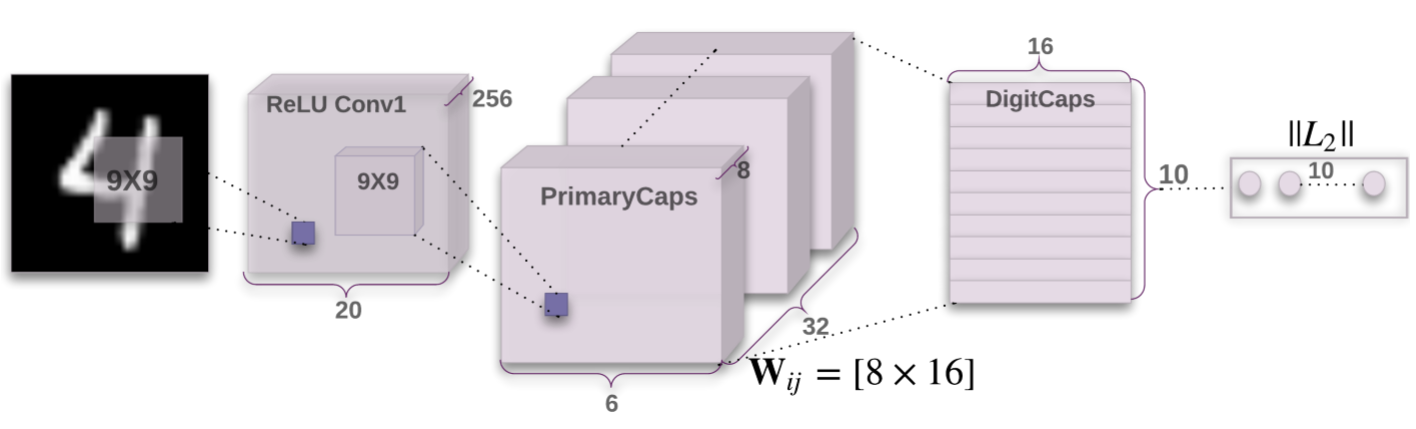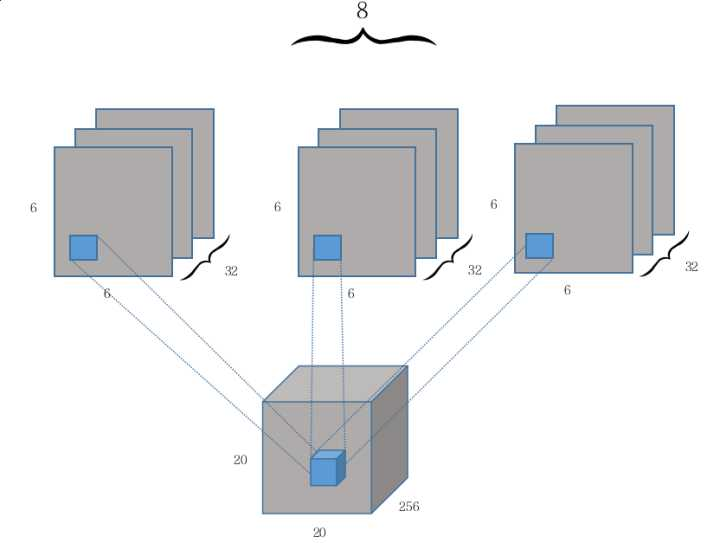PrimaryCaps 每一个向量的分量层级是共享卷积权重的，即获取 6×6 张量的卷积核权重为相同的 9×9 个。这样该卷积层的参数数量为 9×9×256×8×32+8×32=5308672，其中第二部分 8×32 为偏置项参数数量。

DigitCaps 层与 PrimaryCaps 层之间的参数包含两类，即 W_ij 和 c_ij。所有 W_ij 的参数数量应该是 6×6×32×10×8×16=1474560，c_ij 的参数数量为 6×6×32×10×16=184320，此外还应该有 2×1152×10=23040 个偏置项参数，不过原论文并没有明确指出这些偏置项。最后小编计算出该三层 CapsNet 一共有 5537024 个参数，这并不包括后面的全连接重构网络参数。

#### 损失函数与最优化

$L _ { c } = T _ { c } \max \left( 0,m ^ { + } - | \mathbf { v } _ { c } | \right) ^ { 2} + \lambda \left( 1- T _ { c } \right) \max \left( 0,| \mathbf { v } _ { c } | - m ^ { - } \right) ^ { 2}$

#### 重构与表征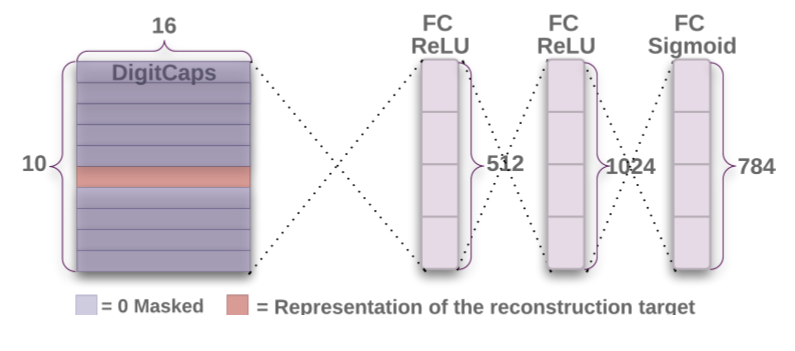Capsule 输出向量的重构与表征除了能提升模型的准确度以外，还能提升模型的可解释性，因为我们能修正需要重构向量中的某个或某些分量而观察重构后的图像变化情况，这有助于我们理解 Capsule 层的输出结果。

### CapsNet 的 TensorFlow 实现